# Introduction

When the response variable is right skewed, many think regression becomes difficult. Skewed data is generally thought of as problematic. However the glm framework provides two options for dealing with right skewed response variables. For the gamma and inverse gaussian distributions, a right skewed response variable is actually helpful.

# Different shapes of a gamma distribution

The critical step is being able to spot a gamma distribution when you see one. Theatrical skewness is $$\frac{2}{\sqrt(shape)}$$. If shape is small, the gamma distribution is right skewed. If shape increases, the gamma becomes more symmetrical

library(GlmSimulatoR)
library(ggplot2)
library(dplyr)
library(stats)

set.seed(1)
#Very right skewed. Skewness 2
Gamma <- rgamma(1000, shape = 1, scale = 1)
temp <- tibble(gamma = Gamma)
ggplot(temp, aes(x=gamma)) +
geom_histogram(bins = 30)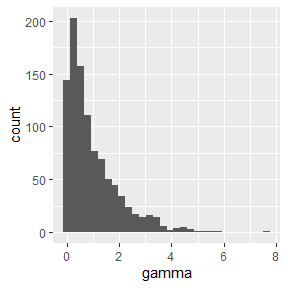#Very right skewed and spread out more. Skewness 2
Gamma <- rgamma(1000, shape = 1, scale = 5)
temp <- tibble(gamma = Gamma)
ggplot(temp, aes(x=gamma)) +
geom_histogram(bins = 30)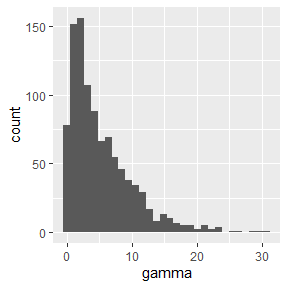#Hump moves slightly towards the middle. Skewness 1.414214
Gamma <- rgamma(1000, shape = 2, scale = 1)
temp <- tibble(gamma = Gamma)
ggplot(temp, aes(x=gamma)) +
geom_histogram(bins = 30)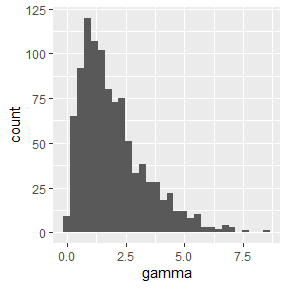#Hump moves slightly more towards the middle. Skewness 1.154701
Gamma <- rgamma(1000, shape = 3, scale = 1)
temp <- tibble(gamma = Gamma)
ggplot(temp, aes(x=gamma)) +
geom_histogram(bins = 30)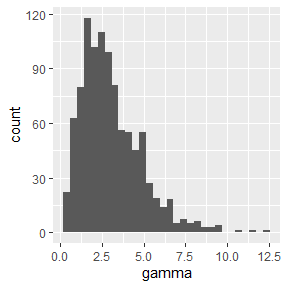#Hump moves slightly more towards the middle. Skewness 0.8944272
Gamma <- rgamma(1000, shape = 5, scale = 1)
temp <- tibble(gamma = Gamma)
ggplot(temp, aes(x=gamma)) +
geom_histogram(bins = 30)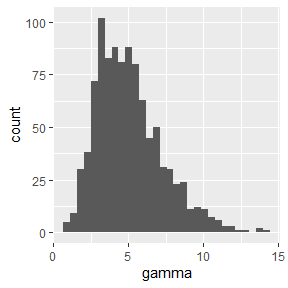#Nearly gaussian. Very slightly right skewed. Skewness .2
Gamma <- rgamma(1000, shape = 100, scale = 1)
temp <- tibble(gamma = Gamma)
ggplot(temp, aes(x=gamma)) +
geom_histogram(bins = 30)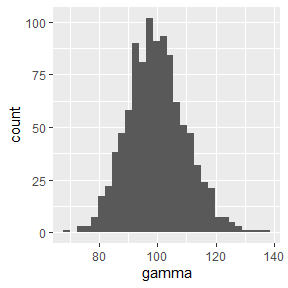# Building models with very skewed data

To show the generalized linear model can handle skewness, lets make some data and train a model and calculate mean squared error.

#Make data
set.seed(1)
simdata <- simulate_gamma(N = 10000, link = "inverse",
weights = c(1, 2, 3), ancillary = .05)
#Confirm Y ~ gamma
ggplot(simdata, aes(x = Y)) +
geom_histogram(bins = 30)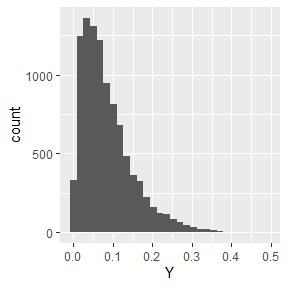glm <- glm(Y ~  X1 + X2 + X3, data = simdata, family = Gamma("inverse"))

#Mean Squared Error
mean((simdata\$Y - predict(glm, newdata = simdata, type = "response"))^2)
#>  0.004147222